IF IT'S EDUCATION, IT'S ITS
Pathways To Learning... Since 2005
Hong Kong Registered School 566985

# I - i to iteration - Mathematics Dictionary

A   B   C   D   E   F   G   H   I   J   K   L   M   N   O   P   Q   R   S   T   U   V   W   X   Y   Z

i: Common symbol used to represent the "positive" square root of -1.

icosagon: A polygon of 20 sides.

icosahedron: A polyhedron of 20 faces. The plural form of icosahedron is icosahedra.

icosidodecahedron: A polyhedron of 32 faces (the word refers to 20 2 10, as in 20 + 12 = 32). The plural form of icosadodecahedron is icosadodecahedra.

ideal point: A point added in turning Euclidean geometry into projective geometry.

idempotent: The property of being unchanged when multiplied by itself. While the identity matrix and the number 1 are trivial examples, the matrix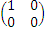are less obvious of an example of an idempotent mathematical object.

identical (of geometric figures): Two shapes are identical (also congruent) if one can be made to coincide with the other through a combination of rotations, reflections and translations.

identity: A mathematical statement with the symbol ≡ that express the equality of the two expressions on either side of it under all circumstances, i.e. no matter what value the variables take. It is a stronger statement than an equation. For example,

x2 - y2 ≡ (x + y)(x – y) See equation.

identity element: For a binary operation, the identity element is the element which, being one of operands involved, leaves the other operand unchanged.

identity function: A function which maps all elements to itself, written f(x) = x.

identity mapping (identity function): A mapping which maps all elements to itself. See monoid.

identity matrix: The matrix multiplicative identity. It is a square matrix whose elements along the main diagonal are all 1 and 0 otherwise.

inferential statistic: A statistic used to describe a population using information from observations on only a probability sample of cases from the population.

Iff common abbreviation for the term "if and only if", used between two mathematical statements to indicate that each is implied by the other, thus their equivalence regardless of the truth value of either statement.

ill-conditioned: The property of a problem where a small change in argument results in a great change to the solution. As a property of the problem, this needs to happen almost nowhere. (i.e. small arguments need to result in great changes everywhere to be considered ill-conditioned, or at least almost everywhere. Similarly, if this happens in only one particular set of arguments, then the problem is not ill-conditioned.)

image: The set of elements in the codomain that corresponds to a specified set of elements in the domain. The image of the domain is sometimes known as the range.

imaginary axis The set of all points representing imaginary numbers, including 0. Commonly the vertical axis in an argand diagram.

imaginary circle: The set of all points satisfying the equation (x - a)2 + (y - b)2 = - r2, where r is a real number. So class="d-title" named because it corresponds with the equation of a circle (x - a)2 + (y - b)2 = r2, with the exception that the part representing the radius now takes an imaginary value.

imaginary number: A complex number whose real part is zero. Imaginary numbers form the set of all square roots of non-positive real numbers.

imaginary part: The number indicating the vertical ordinate in an argand diagram, it is the coefficient of i in a complex number. Alternatively, it is the component of a complex number the square of which is a negative number such that the remaining component (the real part) whose square is a positive number.

imperial units: The system of units used in the British Empire before the adoption of the metric system.

implication: A relation between 2 sentences based on truth values such that it cannot be true that "the antecedent is true and the consequent is false". Usually represented by the symbolsor →.

implicit differentiation: A method of differentiating an implicit equation. One achieves this by differentiating both sides of the equation with respect to one of the variables in the equation, thus maintaining equality in the derivatives. The derivative of one variable with respect to the other can then be found through rearranging the terms.

implicit function: A function defined (and presented) in such a way that there is no clear constructive methods for finding the value of one of its variables through knowledge of the value of the other variables.

improper fraction A fraction where the numerator is greater than or equal to the denominator. In case the numerator and denominator are polynomials in one variables, it is where the degree of the numerator (as a polynomial) is greater than or equal to the degree if the denominator.

improper integral An integral where the concept of infinity is involved in one or both of the limits. It is an improper integral because it is really the limit of a sequence of integrals rather than being an integral itself.

improper rational expression A fraction of polynomials in which the degree in the numerator is higher than that of the denominator. Also called an improper fraction.

impulse: 1. The integral of a (possibly variable) force over time resulting in a change of momentum:Equivalently the total change in momentum of the object upon which the force is applied.

2. The influence which causes the change in momentum.

incentre: The centre of the largest possible circle inscribed in a polygon, known as an incircle.

inch: An imperial unit of length.

incircle The largest possible inscribed circle (usually in a polygon). Similar in form to excircle

inclined plane A plane that is at an angle to the horizontal.

inclusive or: A relation between two sentences where they cannot be both false. (However, they can both be true, or that only one of them is true.) As opposed to exclusive or, where exactly one of the two statements can be true only.

incommensurable: A relation between 2 numbers where their ratio is irrational.

inconsistent equations A system of equations for which it is impossible to find a set of values to satisfy, without assigning different values to the same variable in different equations.

increasing function A function where x > y implies and is implied by f(x) > f(y). Depending on the author, the second inequality may be ≥ so that a increasing function is allowed to "level" as well as increase. In which case, most authors are likely to call the first type strictly increasing functions. Note that monotonic(-ally) increasing functions do not mean that the inquality signs are strict but that the increase is uninterrupted (without exceptions)

increasing sequence A sequence for which each term is not greater than the next. A sequence can be strictly increasing, in which is each term is simply less than the next.

increment An increase in value. Usually taken to mean a "fixed increment", where the amount to be increased by, is always the same.

indefinite integral Also called an antiderivative. The indefinite integral of function f(x) is the (family of) function(s) whose derivative is f(x).

independence The property of two events being independent. Events A and B are independent if the conditional probability of A given B, written P(A|B), equals to P(A), the probability of A alone.

independent equations: 1. A system of equations whose set of solutions is not a subset of another system of equations.

2. A system of equations where all partitions of the equations have one subset being independent (in the sense of 1) to another (non-intersecting) subset.

independent events Two events are independent if their outcomes are unaffected by the other.

independent variable: A variable considered not to be a function of another variable (or set of variables) within the system being considered.

indeterminate equation An equation that is seen (depending on the domain) to support all or almost all possible solutions. Note that this concept is not a precise one, since polynomials can have an arbitrarily large number of solutions, while trigonometric equations supports infinitely many solutions, even though they're not usually considered indeterminate.

indeterminate expression A expression having no definitive value assigned, yet could be seen to take on various values in different circumstances. e.g. depending on the function used, f(x)=0/x or f(x)=x/x, the expression 0/0 can be seen to take on the value of 0 or 1 respectively, assuming that it is consistent with the limits, yet the two limits are not the same. Thus 0/0 is seen as indeterminate.

index(plural indices): 1. (index number) A measure of change of the value of some variable relative to some reference point in (usually) time, that is usually defined such that the number (index) is normalised to reflect some powers of 10 at the reference point.

2.Another class="d-title" name for an exponent or "power".

index notation: 1. A method for denoting the elements of a array of mathematical objects.

2. The method of representing exponentations through the use of superscripts.

index set A set of elements (usually but not limited to numbers) that is used to map (usually) bijectively to a set of objects of interest so that the objects of interest can be indentified through the index elements. e.g. The set {1,2,3,4} is the index set of the set of variables {x1, x2, x3, x4}

indicator diagram: A type of diagrams used in engineering to measure the work done through the area in the diagram.

indicator function A function defined for a set X of objects of interest such that it returns the number 1 for objects in X within a defined subset of Y and return 0 otherwise. It can sometimes be used to define a subset instead.

indices: Plural of the word index. Indexes is also used, especially in the first sense of the word index.

indirect proof Also called a proof by contradiction or reductio ad absurdum. It works by demonstrating that the EXACT opposite to your point of view MUST lead to a contradiction, thereby yours must be the only valid viewpoint. This method dismisses para-consistency logic and assumes the Law of Excluded Middle.

individual constant: A class="d-title" name for an individual (in the sense of formal logic) on which quantifiers cannot apply.

induction A method of proving a class of statements where the statements are well-ordered by establishing that the first statement is true (the base case) and that a statement within the class being true implies that the next statement (within the well-ordering) in true (the inductive step.).

inelastic collision: A collision of 2 bodies where kinetic energy is lost (to heat etc.).

inequality: Any mathematical sentences that states the relationship between 2 values or expressions by asserting that:

1) They are not the same

2) That one is greater than the other. / That one is less than the other.

3) That one is not greater than the other. / That one is not less than the other.

Through the use of symbols ≠, >, ≥, < or≤.

inertial coordinate system: A coordinate system where the velocity of any objects in uniform motion is a constant vector within the system.

inertial frame of reference: An inertial coordinate system for positions and related concepts.

inertial mass: The measure of the (un)willingness of a physical object to acclerate.

inessential map: In topology, the morphism for a homotopy which maps the fundamental class to zero.

Inf: A shorthand for infimum.

infimum: A greatest lower bound (of a set).

infinite decimal Also called nonterminating decimal

infinite discontinuity: A discontinuity of a function where at least one of the (left-hand and right-hand) limits is infinite.

infinite group: A group with infinitely many elements.

infinite integral: Also known as an improper integral, it is where an integral involves infinity in either its limits (upper or lower or both)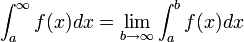or the values of the integrandinfinite limit: A limit that is unbounded.

infinite product: A multiplication equivalent for infinite series, denoted by the capital letter for π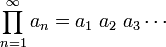infinite sequence A sequence with infinitely many terms.

infinite series A series with infinitely many terms.

infinitesimal An infinitely small quantity. A quantity that is greater than zero but smaller than all positive values. It is understood in terms of limits, or in the case of non-standard analysis, in a more literal sense.

infinitesimal calculus: A term for the branch of mathematics usually known simply as calculus. This term is necessary since the term is calculus is also associated with other unrelated fields in mathematics (e.g. lambda calculus, which is not about derivatives and integrals.)

infinity: Informally, a quantity without bound represented by the symbol ∞. It can also be made more precise in set theory as a set which is bijective with a subset of itself, where the definition can filter down to other fields in mathematics.

inflection: A point ot the generall process of changing from one type of curavture to the other (i.e. concavity and convexity).

initial conditions: A type of boundary condition specifically giving information of the system at the beginning of the time interval in consideration.

injection A function where distinct arguments always yields distinct values.

inner product: A generalisation of the scalar product for abstract vector spaces.

inradius: The radius of the largest possible circle inscribed in a polygon, known as an incircle.

inscribed: A shape which is the largest (given the specified parameters, usually shape) inside another.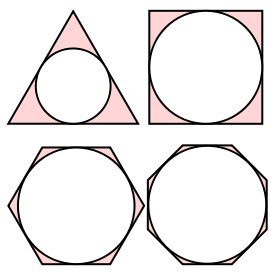instantaneous: Related to only one point in time. (Or similar independent variables, by extension.)

instanton: Also known as a pseudo-particle.

integer: The set of all counting numbers (natural numbers), their additive inverses (i.e. the negative equivalents), and 0. Usually denited by a bold (or blackboard-bold) Z.

integer lattice: A subset of the Euclidean space Rn considered as a group consisting of points whose coordinates are all integers.

integrability The property where an expression can be integrated.

integrable An integrable function is a function that can be integrated.

integral: 1. The resulting expression of an integration.

2. Having the property of relating to or being an integer.

integral calculus: One of two main parts of calculus which is concerned with the study of integrals, as opposed to differential calculus.

integral of a power series: An alternative method of integration of a function by integrating the power series representing the function, given that the function (the integrand) can be represented by a power series.

integral sign: The symbol ∫ used to represent integration - the symbol originates from considering integration as a modified (infintie) form of summation, which uses a large capital sigma in a similar capacity.

integrand The expression in an integral that is to be integrated.

integrating factor An expression that both side of a first order linear differential equations are multiplied by so that both sides become exact differentials, facilitating an easier integration process.

integration: The inverse process of differentiation, as related by the fundamental theorem of calculus. It can informally thought of as the process of finding functions (called integrals) which calculates the limit of sums of (different) constant quantities over a number of small intervals for what is actually a variable quantity over an interval.

integration by partial fractions A method for easily integrating rational functions by splitting it up into fractions with simpler denominators. (By considering complex numbers the denominator can be split into linear factors, and thus, fractions all consisting of exactly one linear factor.)

integration by parts: A method of integrating a product, by designating one half of the product to be eventually integrated while the other half differentiated, following the ruleintegration by substitution A method akin to, but weaker than, the chain rule of differentiation, that allows the integration of an expression by a change of variables.

interaction: The relation between variables in statistics where the influence on a variable from a set of variables is such that the total effect is not simply a sum of the effects from the individual variables.

intercept The intersection of a line/plane with another line/plane.

intercept form: The expression of an object such as a line, a plane or higher dimensional equivalents in equations in a form which clear shows the object's intersection with coordinate axes, or planes and higher dimensional equivalents formed from these axes.

intercept theorem: A theorem regarding ratios in similar triangles differing in its construction by intersecting a pair of parallel lines with two lines which intersect each other.interest: A fee paid for use of an asset (often money) without taking ownership (the asset must be returned "as is" at the time of borrowing/lending).

interior The points within the bounded section of a geometric figure.

interior angle The amount of rotation, centring on the vertex, of one edge of a polygon in order to coincide with an adjacent edge, through only the interior of the polygon.

internal force: A force on a part of the system originating from another part of the system under consideration. As opposed to external force.

internal tangent: The relation between two circles which intersect at exactly one point where the centres of at least one of the circles is within the other circle (it is also possible that both is true). As opposed to external tangent.

interpolation: Constructing new values by use of existing values where the argument of the new construction is between those of the existing values. As opposed to extrapolation.

interquartile range A measure of dispersion of a sample, defined to be the difference between the lower and upper quartiles. The related measure of the semi-interquartile range is simply half this difference.

intersection: 1. The set whose elements are elements of both sets in question. (Lines, planes and other geometric figures, as well as more abstract sets.)

2. The class="d-title" name of the operation and operator related to creating a new set which is the intersection of 2 existing sets.

interval A set of real numbers such that any number between two numbers in the set is also in the set.

interval estimate: The estimate of a (unknown) value by giving an interval of values. As opposed to giving a value, called point estimate.

interval of convergence: An interval on real numbers within which arguments produce a limit (i.e. the sum is convergent) in a given series.

interval scale: A system of representation where the difference between 2 quantities is directly proportional to the distance between the representation of the 2 objects, where the proportion (called the scale) is known and units of distances often marked.

intransitive relation: A relation which is not transitive.

intrinsic equation: A way of defining a curve using intrinsic properties of curves such as arc lengths and curvatures.invariance The property that a quantity is unchanged (under some transformations) or quantites exhibiting such properties.

invariant Describing the property that a quantity is unchanged (under some transformations).

inverse: An object of the same type as one given that involves a juxtaposition of how it relates to other objects (of any types), e.g.

1. function: juxtaposition of inputs and outputs of a given function

2. matrix: juxtaposition of multiplicand and product of a given matrix

3. number (additive): counterpart in the juxtaposition of addends to form a sum of zero

4. number (multiplicative): juxtaposition of multiplicand and product (also called a reciprocal)

5. Transformation: juxtaposition of the argument (geometric figure input, i.e. before) and value (geometric figure output, i.e. after).

Note that the juxtaposition of antecedent and consequent in a sentence involving logical implication is actually called a converse. The inverse of a statement is one which replaces both antecedent and consequent with their negation.

inverse hyperbolic functions: The functions which take the values of hyperbolic functions as argument and give the corresponding arguments of the same function as values.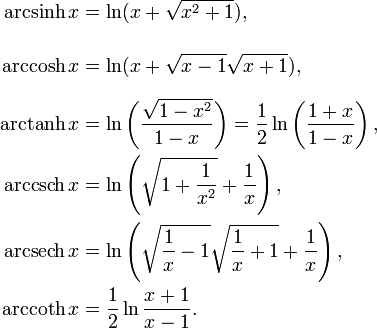inverse of a conditional: The sentence formed by replacing both the antecedent and the consequent with their respective negation from a given sentence involving logical implication.

inversely proportional: The relation between 2 variable quantities where each is proportional to the reciprocal of the other.

inverse ratio: The ratio of the reciprocals of 2 given quantities.

inverse sine series: The Maclaurin series expansion for arcsin x (the function inverse sine):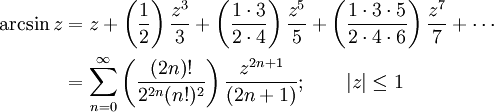inverse square law: Any laws (often related to the physical world) where one quantity is inversely proportional to the square of the other. e.g. a law between magitude of gravitation between two masses and their distance.

inverse trigonometric functions: The functions which take the values of trigonometric functions as argument and give the corresponding arguments of the same function as values. Examples of inverse trigonometric fnctions are arcsin x, arccos x, arctan x, arccot x etc.

inverse variation: Also known as inverse proportions.

inversion: A transformation where a point is mapped to another point such that the centre of a reference circle form a straight line with the two points (a point and its image), without the centre in between and such that the geometric mean of the distances of the centre from the two points is the radius of the circle..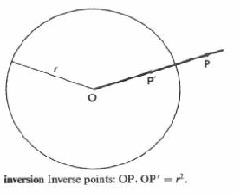invertible Describing the property of having an inverse.

involute: The locus of points traced from a variable point on the variable tangent of a reference curve with a distance from the point of contact the same as the arc length between the contact point and a reference point, in the same "direction" from the contact point as the reference point.

involution: 1. The process of raising a number or expression to a certain power.

2. A self-inverse function or matrix.

involutory: Of being an involution.

IVP: Initial value problem - an ordinary differential equation with initial conditions.

irrational number: A number which is not rational.

irreducible equation: An equation formed by an irreducible polynomial.

irreducible fraction: A fraction most simplified, such that the numerators and denominators of any equivalent fractions are greater than the ones used.

irreducible polynomial: A polynomial which cannot be further factorised given the (field of) coefficients under consideration.

irreflexive relation: A relation that is not reflexive.

irrotational vector: Mathematically often called an irrotational vector field, it is a vector field whose curl (a vector differential operator) is identically zero is some specified region.

isochrone: Also known as a tautochrone, the curve where the time particles take in reaching the minimum by sliding under gravity without friction is independent of their starting positions.

isogonal: General term for equiangular in all dimensions.

isolate: 1. The process of attending to a particular mathematical object over others of the same type, set or family.

2. The process of giving the value of a root to a certain accuracy by considering values on either side of the (propsed) root in a continuous function (often a polynomial).

isolated point: Also known as a hermit point or acnode, a solution to a system of equations where the solution (amongst uncountably many) have no other solutions within its neighbourhood. e.g. y2 = sec x + 1 has (countably) infinitely many isolated points.

isolated singularity: A singular point with no other singular points in its neighbourhood.

isometry (isometric map) A transformation where distances between two points are invariant. Any combinations of translations, rotations and reflections are isometries.

isoperimetric The property of having equal perimeters.

isoperimetric inequality An inequality stating the relationship between the perimeter and area of a closed plane curve. Note that a circle satisfy the equality part of this statement.

isosceles trapezium A trapezium with its opposite non-parallel sides equal in length.

isosceles triangle A triangle with two sides equal in length and equivalently, two angles being equal.

iterated integral: An expression with nested integrals, also known as multiple integration.

iteration The process of repeating a set of instructions by using the output from one implementation of the instructions as the input for the next implementation.

A   B   C   D   E   F   G   H   I   J   K   L   M   N   O   P   Q   R   S   T   U   V   W   X   Y   Z

Genius is one percent inspiration and ninety-nine percent perspiration.